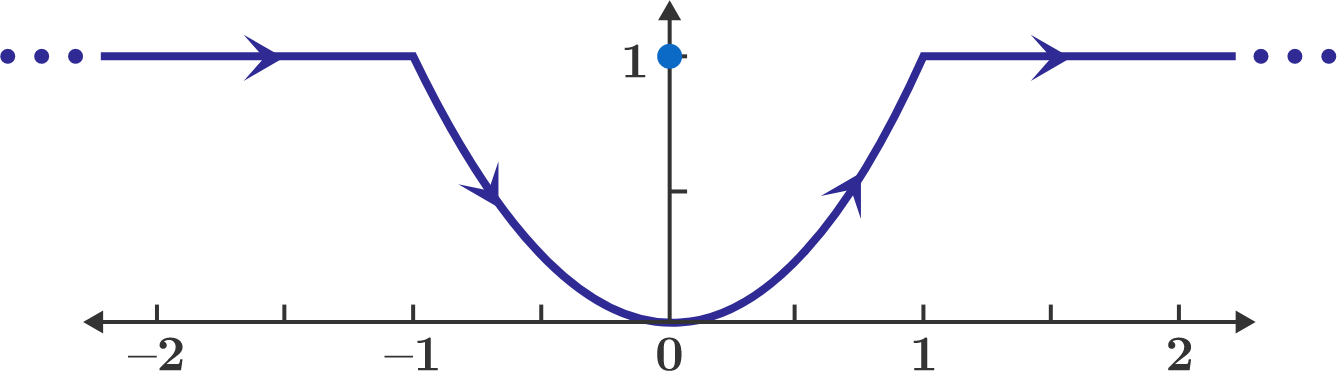# Parabola Magnetic Field

A wire in the shape of the curve $y = x^2$ carries a current $I.$ It also contains some horizontal, semi-infinite segments.

The magnitude of the magnetic flux density $(B)$ at the point $(x,y) = (0,1)$ is $B = \alpha \, \frac{\mu_0 \, I}{4 \pi}.$ If $\alpha$ is a positive real number, what is its value?×